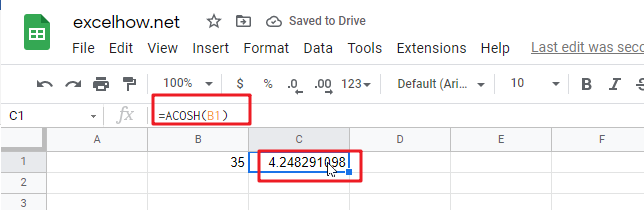# ExcelHow

This post will guide you how to use Google Sheets ACOSH function with syntax and examples.

## Description

The Google Sheets ACOSH function returns the inverse hyperbolic cosine of a number.

The ACOSH function is a build-in function in Google Sheets and it is categorized as a MATH function.

## Syntax

The syntax of the ACOSH function is as below:

= ACOSH (number)

Where the ACOSH function argument is:

• Number – This is a required argument.  Any real number greater than or equal to 1.

## Google Sheets ACOSH Function Examples

The below example will show you how to use google sheets ACOSH function to return the inverse hyperbolic cosine of the B1 cell.

#1 =ACOSH(B1)